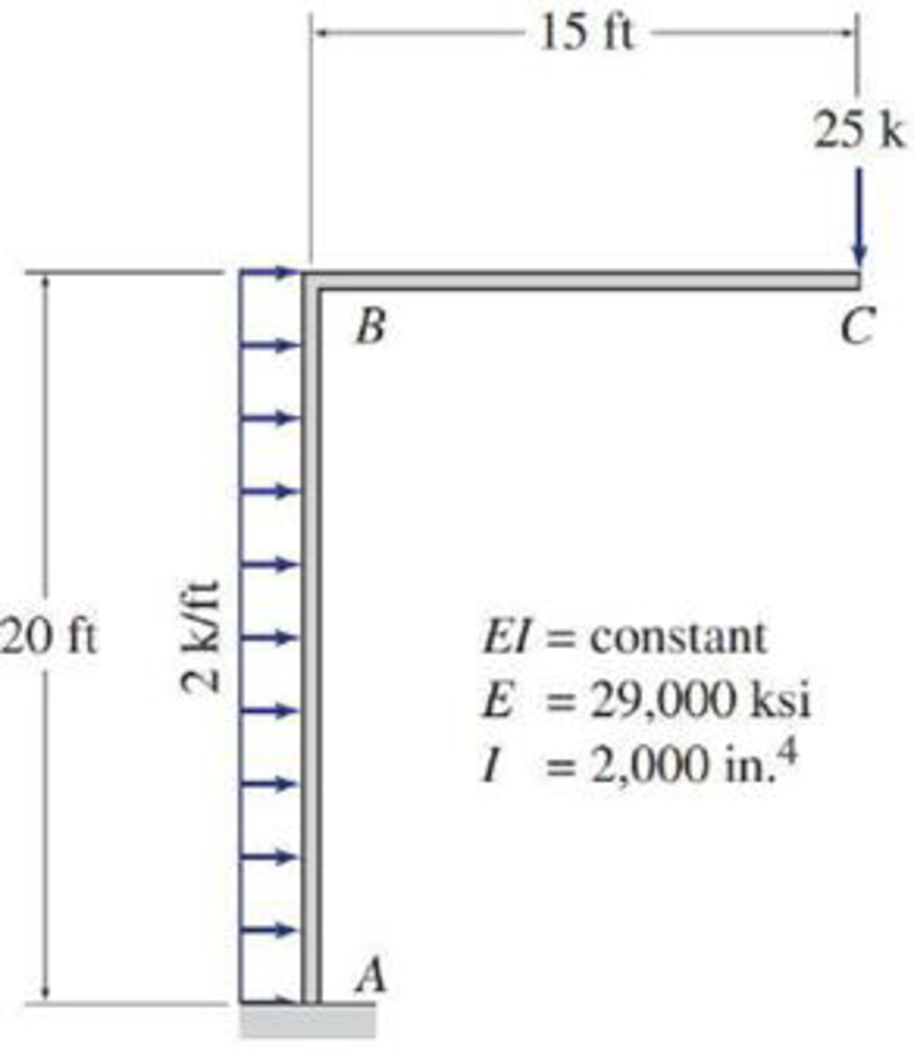# 7.36 and 7.37 Use the virtual work method to determine the vertical deflection at joint C of the frame shown.

#### Solutions

Chapter
Section
Chapter 7, Problem 36P
Textbook Problem
289 views

## 7.36 and 7.37 Use the virtual work method to determine the vertical deflection at joint C of the frame shown.To determine

Find the vertical deflection at joint C of the frame using virtual work method.

### Explanation of Solution

Given information:

The frame is given in the Figure.

The value of E is 29,000 ksi and I is 2000in.4.

Apply the sign conventions for calculating reactions, forces and moments using the three equations of equilibrium as shown below.

• For summation of forces along x-direction is equal to zero (Fx=0), consider the forces acting towards right side as positive (+) and the forces acting towards left side as negative ().
• For summation of forces along y-direction is equal to zero (Fy=0), consider the upward force as positive (+) and the downward force as negative ().
• For summation of moment about a joint is equal to zero (Matapoint=0), consider the clockwise moment as negative and the counter clockwise moment as positive.

Calculation:

Consider the real system.

Draw a diagram showing all the given real loads acting on it.

Let the bending moment due to real load be M.

Sketch the real system of the frame as shown in Figure 1.

Find the reactions and moment at the supports A:

Summation of moments about A is equal to 0.

MA=0MA25(15)2(20)(202)=0MA=775k-ft

Summation of forces along y-direction is equal to 0.

+Fy=0Ay25=0Ay=25k

Summation of forces along x-direction is equal to 0.

+Fx=0Ax+2(20)=0Ax=20k

Consider the virtual system.

Draw a diagram of frame without the given real loads. For vertical deflection apply unit load at the joint in the vertical direction.

Let the bending moment due to virtual load be Mv.

Sketch the virtual system of the frame with unit load at joint C as shown in Figure 2.

Find the reactions and moment at the supports A:

Summation of moments about A is equal to 0.

MA=0MA1(15)=0MA=15k-ft

Summation of forces along y-direction is equal to 0.

+Fy=0Ay1=0Ay=1k

Find the equations for M and Mv for the 2 segments of the frame as shown in Table 1.

 Segment x-coordinate M (k-ft) Mv (k-ft) Origin Limits (ft) BA B 0−20 375+x2 15 CB C 0−15 −25x −x

Find the deflection at C using the virtual work expression:

1(ΔC)=0LMvMEIdx (1)

Rearrange Equation (1) for the limits 020 and 015 as follows

### Still sussing out bartleby?

Check out a sample textbook solution.

See a sample solution

#### The Solution to Your Study Problems

Bartleby provides explanations to thousands of textbook problems written by our experts, many with advanced degrees!

Get Started

Find more solutions based on key concepts
In your own words, explain the difference between absolute humidity and relative humidity.

Engineering Fundamentals: An Introduction to Engineering (MindTap Course List)

Explain how to use a push stick.

Precision Machining Technology (MindTap Course List)

How is above base speed obtained?

Electric Motor Control

Principles of Information Systems (MindTap Course List)

What types of files might you want to transfer between your computer and mobile device using Bluetooth?

Enhanced Discovering Computers 2017 (Shelly Cashman Series) (MindTap Course List)

How can security be both a project and a process?

Management Of Information Security

Explain Appleâ€™s view of user interface design, especially for apps.

Systems Analysis and Design (Shelly Cashman Series) (MindTap Course List)

What problem can occur if welding machines are stacked or placed too closely together?

Welding: Principles and Applications (MindTap Course List)

If your motherboard supports ECC DDR3 memory, can you substitute non-ECC DDR3 memory?

A+ Guide to Hardware (Standalone Book) (MindTap Course List)